Professor Murray

Sine and Cosine Functions

Slide Duration:

Section 1: Trigonometric Functions
Angles

39m 5s

Intro
0:00
Degrees
0:22
Circle is 360 Degrees
0:48
Splitting a Circle
1:13
2:08
2:31
2:52
Half-Circle and Right Angle
4:00
6:24
6:52
Coterminal, Complementary, Supplementary Angles
7:23
Coterminal Angles
7:30
Complementary Angles
9:40
Supplementary Angles
10:08
Example 1: Dividing a Circle
10:38
Example 2: Converting Between Degrees and Radians
11:56
Example 3: Quadrants and Coterminal Angles
14:18
Extra Example 1: Common Angle Conversions
-1
Extra Example 2: Quadrants and Coterminal Angles
-2
Sine and Cosine Functions

43m 16s

Intro
0:00
Sine and Cosine
0:15
Unit Circle
0:22
Coordinates on Unit Circle
1:03
Right Triangles
1:52
2:25
Master Right Triangle Formula: SOHCAHTOA
2:48
Odd Functions, Even Functions
4:40
Example: Odd Function
4:56
Example: Even Function
7:30
Example 1: Sine and Cosine
10:27
Example 2: Graphing Sine and Cosine Functions
14:39
Example 3: Right Triangle
21:40
Example 4: Odd, Even, or Neither
26:01
Extra Example 1: Right Triangle
-1
Extra Example 2: Graphing Sine and Cosine Functions
-2
Sine and Cosine Values of Special Angles

33m 5s

Intro
0:00
45-45-90 Triangle and 30-60-90 Triangle
0:08
45-45-90 Triangle
0:21
30-60-90 Triangle
2:06
Mnemonic: All Students Take Calculus (ASTC)
5:21
Using the Unit Circle
5:59
New Angles
6:21
9:43
Mnemonic: All Students Take Calculus
10:13
13:11
16:48
Example 3: All Angles and Quadrants
20:21
Extra Example 1: Convert, Quadrant, Sine/Cosine
-1
Extra Example 2: All Angles and Quadrants
-2
Modified Sine Waves: Asin(Bx+C)+D and Acos(Bx+C)+D

52m 3s

Intro
0:00
Amplitude and Period of a Sine Wave
0:38
Sine Wave Graph
0:58
Amplitude: Distance from Middle to Peak
1:18
Peak: Distance from Peak to Peak
2:41
Phase Shift and Vertical Shift
4:13
Phase Shift: Distance Shifted Horizontally
4:16
Vertical Shift: Distance Shifted Vertically
6:48
Example 1: Amplitude/Period/Phase and Vertical Shift
8:04
Example 2: Amplitude/Period/Phase and Vertical Shift
17:39
Example 3: Find Sine Wave Given Attributes
25:23
Extra Example 1: Amplitude/Period/Phase and Vertical Shift
-1
Extra Example 2: Find Cosine Wave Given Attributes
-2
Tangent and Cotangent Functions

36m 4s

Intro
0:00
Tangent and Cotangent Definitions
0:21
Tangent Definition
0:25
Cotangent Definition
0:47
Master Formula: SOHCAHTOA
1:01
Mnemonic
1:16
Tangent and Cotangent Values
2:29
Remember Common Values of Sine and Cosine
2:46
90 Degrees Undefined
4:36
Slope and Menmonic: ASTC
5:47
Uses of Tangent
5:54
Example: Tangent of Angle is Slope
6:09
7:49
Example 1: Graph Tangent and Cotangent Functions
10:42
Example 2: Tangent and Cotangent of Angles
16:09
Example 3: Odd, Even, or Neither
18:56
Extra Example 1: Tangent and Cotangent of Angles
-1
Extra Example 2: Tangent and Cotangent of Angles
-2
Secant and Cosecant Functions

27m 18s

Intro
0:00
Secant and Cosecant Definitions
0:17
Secant Definition
0:18
Cosecant Definition
0:33
Example 1: Graph Secant Function
0:48
Example 2: Values of Secant and Cosecant
6:49
Example 3: Odd, Even, or Neither
12:49
Extra Example 1: Graph of Cosecant Function
-1
Extra Example 2: Values of Secant and Cosecant
-2
Inverse Trigonometric Functions

32m 58s

Intro
0:00
Arcsine Function
0:24
Restrictions between -1 and 1
0:43
Arcsine Notation
1:26
Arccosine Function
3:07
Restrictions between -1 and 1
3:36
Cosine Notation
3:53
Arctangent Function
4:30
Between -Pi/2 and Pi/2
4:44
Tangent Notation
5:02
Example 1: Domain/Range/Graph of Arcsine
5:45
Example 2: Arcsin/Arccos/Arctan Values
10:46
Example 3: Domain/Range/Graph of Arctangent
17:14
Extra Example 1: Domain/Range/Graph of Arccosine
-1
Extra Example 2: Arcsin/Arccos/Arctan Values
-2
Computations of Inverse Trigonometric Functions

31m 8s

Intro
0:00
Inverse Trigonometric Function Domains and Ranges
0:31
Arcsine
0:41
Arccosine
1:14
Arctangent
1:41
Example 1: Arcsines of Common Values
2:44
Example 2: Odd, Even, or Neither
5:57
Example 3: Arccosines of Common Values
12:24
Extra Example 1: Arctangents of Common Values
-1
Extra Example 2: Arcsin/Arccos/Arctan Values
-2
Section 2: Trigonometric Identities
Pythagorean Identity

19m 11s

Intro
0:00
Pythagorean Identity
0:17
Pythagorean Triangle
0:27
Pythagorean Identity
0:45
Example 1: Use Pythagorean Theorem to Prove Pythagorean Identity
1:14
Example 2: Find Angle Given Cosine and Quadrant
4:18
Example 3: Verify Trigonometric Identity
8:00
Extra Example 1: Use Pythagorean Identity to Prove Pythagorean Theorem
-1
Extra Example 2: Find Angle Given Cosine and Quadrant
-2
Identity Tan(squared)x+1=Sec(squared)x

23m 16s

Intro
0:00
Main Formulas
0:19
Companion to Pythagorean Identity
0:27
For Cotangents and Cosecants
0:52
How to Remember
0:58
Example 1: Prove the Identity
1:40
Example 2: Given Tan Find Sec
3:42
Example 3: Prove the Identity
7:45
Extra Example 1: Prove the Identity
-1
Extra Example 2: Given Sec Find Tan
-2

52m 52s

Intro
0:00
0:09
How to Remember
0:48
Cofunction Identities
1:31
How to Remember Graphically
1:44
Where to Use Cofunction Identities
2:52
Example 1: Derive the Formula for cos(A-B)
3:08
Example 2: Use Addition and Subtraction Formulas
16:03
Example 3: Use Addition and Subtraction Formulas to Prove Identity
25:11
Extra Example 1: Use cos(A-B) and Cofunction Identities
-1
Extra Example 2: Convert to Radians and use Formulas
-2
Double Angle Formulas

29m 5s

Intro
0:00
Main Formula
0:07
How to Remember from Addition Formula
0:18
Two Other Forms
1:35
Example 1: Find Sine and Cosine of Angle using Double Angle
3:16
Example 2: Prove Trigonometric Identity using Double Angle
9:37
Example 3: Use Addition and Subtraction Formulas
12:38
Extra Example 1: Find Sine and Cosine of Angle using Double Angle
-1
Extra Example 2: Prove Trigonometric Identity using Double Angle
-2
Half-Angle Formulas

43m 55s

Intro
0:00
Main Formulas
0:09
Confusing Part
0:34
Example 1: Find Sine and Cosine of Angle using Half-Angle
0:54
Example 2: Prove Trigonometric Identity using Half-Angle
11:51
Example 3: Prove the Half-Angle Formula for Tangents
18:39
Extra Example 1: Find Sine and Cosine of Angle using Half-Angle
-1
Extra Example 2: Prove Trigonometric Identity using Half-Angle
-2
Section 3: Applications of Trigonometry
Trigonometry in Right Angles

25m 43s

Intro
0:00
Master Formula for Right Angles
0:11
SOHCAHTOA
0:15
Only for Right Triangles
1:26
Example 1: Find All Angles in a Triangle
2:19
Example 2: Find Lengths of All Sides of Triangle
7:39
Example 3: Find All Angles in a Triangle
11:00
Extra Example 1: Find All Angles in a Triangle
-1
Extra Example 2: Find Lengths of All Sides of Triangle
-2
Law of Sines

56m 40s

Intro
0:00
Law of Sines Formula
0:18
SOHCAHTOA
0:27
Any Triangle
0:59
Graphical Representation
1:25
Solving Triangle Completely
2:37
When to Use Law of Sines
2:55
ASA, SAA, SSA, AAA
2:59
SAS, SSS for Law of Cosines
7:11
Example 1: How Many Triangles Satisfy Conditions, Solve Completely
8:44
Example 2: How Many Triangles Satisfy Conditions, Solve Completely
15:30
Example 3: How Many Triangles Satisfy Conditions, Solve Completely
28:32
Extra Example 1: How Many Triangles Satisfy Conditions, Solve Completely
-1
Extra Example 2: How Many Triangles Satisfy Conditions, Solve Completely
-2
Law of Cosines

49m 5s

Intro
0:00
Law of Cosines Formula
0:23
Graphical Representation
0:34
Relates Sides to Angles
1:00
Any Triangle
1:20
Generalization of Pythagorean Theorem
1:32
When to Use Law of Cosines
2:26
SAS, SSS
2:30
Heron's Formula
4:49
Semiperimeter S
5:11
Example 1: How Many Triangles Satisfy Conditions, Solve Completely
5:53
Example 2: How Many Triangles Satisfy Conditions, Solve Completely
15:19
Example 3: Find Area of a Triangle Given All Side Lengths
26:33
Extra Example 1: How Many Triangles Satisfy Conditions, Solve Completely
-1
Extra Example 2: Length of Third Side and Area of Triangle
-2
Finding the Area of a Triangle

27m 37s

Intro
0:00
Master Right Triangle Formula and Law of Cosines
0:19
SOHCAHTOA
0:27
Law of Cosines
1:23
Heron's Formula
2:22
Semiperimeter S
2:37
Example 1: Area of Triangle with Two Sides and One Angle
3:12
Example 2: Area of Triangle with Three Sides
6:11
Example 3: Area of Triangle with Three Sides, No Heron's Formula
8:50
Extra Example 1: Area of Triangle with Two Sides and One Angle
-1
Extra Example 2: Area of Triangle with Two Sides and One Angle
-2
Word Problems and Applications of Trigonometry

34m 25s

Intro
0:00
Formulas to Remember
0:11
SOHCAHTOA
0:15
Law of Sines
0:55
Law of Cosines
1:48
Heron's Formula
2:46
Example 1: Telephone Pole Height
4:01
Example 2: Bridge Length
7:48
Example 3: Area of Triangular Field
14:20
Extra Example 1: Kite Height
-1
Extra Example 2: Roads to a Town
-2
Vectors

46m 42s

Intro
0:00
Vector Formulas and Concepts
0:12
Vectors as Arrows
0:28
Magnitude
0:38
Direction
0:50
Drawing Vectors
1:16
Uses of Vectors: Velocity, Force
1:37
Vector Magnitude Formula
3:15
Vector Direction Formula
3:28
Vector Components
6:27
Example 1: Magnitude and Direction of Vector
8:00
Example 2: Force to a Box on a Ramp
12:25
Example 3: Plane with Wind
18:30
Extra Example 1: Components of a Vector
-1
Extra Example 2: Ship with a Current
-2
Section 4: Complex Numbers and Polar Coordinates
Polar Coordinates

1h 7m 35s

Intro
0:00
Polar Coordinates vs Rectangular/Cartesian Coordinates
0:12
Rectangular Coordinates, Cartesian Coordinates
0:23
Polar Coordinates
0:59
Converting Between Polar and Rectangular Coordinates
2:06
R
2:16
Theta
2:48
Example 1: Convert Rectangular to Polar Coordinates
6:53
Example 2: Convert Polar to Rectangular Coordinates
17:28
Example 3: Graph the Polar Equation
28:00
Extra Example 1: Convert Polar to Rectangular Coordinates
-1
Extra Example 2: Graph the Polar Equation
-2
Complex Numbers

35m 59s

Intro
0:00
Main Definition
0:07
Number i
0:23
Complex Number Form
0:33
Powers of Imaginary Number i
1:00
Repeating Pattern
1:43
Operations on Complex Numbers
3:30
3:39
Multiplying Complex Numbers
4:39
FOIL Method
5:06
Conjugation
6:29
Dividing Complex Numbers
7:34
Conjugate of Denominator
7:45
Example 1: Solve For Complex Number z
11:02
Example 2: Expand and Simplify
15:34
Example 3: Simplify the Powers of i
17:50
Extra Example 1: Simplify
-1
Extra Example 2: All Complex Numbers Satisfying Equation
-2
Polar Form of Complex Numbers

40m 43s

Intro
0:00
Polar Coordinates
0:49
Rectangular Form
0:52
Polar Form
1:25
R and Theta
1:51
Polar Form Conversion
2:27
R and Theta
2:35
Optimal Values
4:05
Euler's Formula
4:25
Multiplying Two Complex Numbers in Polar Form
6:10
Multiply r's Together and Add Exponents
6:32
Example 1: Convert Rectangular to Polar Form
7:17
Example 2: Convert Polar to Rectangular Form
13:49
Example 3: Multiply Two Complex Numbers
17:28
Extra Example 1: Convert Between Rectangular and Polar Forms
-1
Extra Example 2: Simplify Expression to Polar Form
-2
DeMoivre's Theorem

57m 37s

Intro
0:00
Introduction to DeMoivre's Theorem
0:10
n nth Roots
3:06
DeMoivre's Theorem: Finding nth Roots
3:52
Relation to Unit Circle
6:29
One nth Root for Each Value of k
7:11
Example 1: Convert to Polar Form and Use DeMoivre's Theorem
8:24
Example 2: Find Complex Eighth Roots
15:27
Example 3: Find Complex Roots
27:49
Extra Example 1: Convert to Polar Form and Use DeMoivre's Theorem
-1
Extra Example 2: Find Complex Fourth Roots
-2
Bookmark & Share Embed

## Copy & Paste this embed code into your website’s HTML

Please ensure that your website editor is in text mode when you paste the code.
(In Wordpress, the mode button is on the top right corner.)
×
• - Allow users to view the embedded video in full-size.
Since this lesson is not free, only the preview will appear on your website.

• ## Related Books1 answerLast reply by: Dr. Will MurrayMon Jun 15, 2020 10:24 AMPost by Victoria Su on June 13, 2020HiFor example 4, I understand  why they odd/even based on the graph. However,  I am not too sure how it works with plugging in the x values for f(x ) or g(x). I tried plugging them in but didn't get the correct answer.1 answerLast reply by: Dr. Will MurrayFri Apr 3, 2020 10:03 AMPost by Cheung Chun Hei on April 1, 2020I still don't get the part about whether the graph is rotational or mirror symmetry and whether it is odd or even. Can you explain this a bit and thank you so much!1 answerLast reply by: Dr. Will MurrayFri Mar 27, 2020 9:55 AMPost by Yanping Du on March 25, 2020What happens when a graph is both mirror symmetric and rotationally symmetric? Is that possible?1 answerLast reply by: Dr. William MurraySun Mar 8, 2020 10:58 AMPost by susiexuthus on March 7, 2020When I try to load the extra examples, it says in the bottom right corner "audio source file does not exist". Is it just my  computer?1 answerLast reply by: Dr. William MurrayWed Apr 17, 2019 1:35 PMPost by Christopher Wang on April 16, 2019I don't understand how you derived the fact that cos pi is -1 and sin pi is 0.1 answerLast reply by: Dr. William MurrayTue Jun 7, 2016 12:31 PMPost by Bassam Razzaq on June 5, 2016In example 2, how can the graph has value of x-coordinate more than 2pi. I thought 2pi was the last point as also seen in unit circle.1 answerLast reply by: Dr. William MurrayMon Nov 2, 2015 2:18 PMPost by Peter Ke on October 30, 2015In example 4, I DON'T get how g(x) is odd. I thought it was even.3 answersLast reply by: Dr. William MurrayWed Nov 11, 2015 9:23 AMPost by Peter Ke on October 30, 2015For example 3 shouldn't Sin = 4/5 be 3/5? Because 3 is the opposite and 4 is adjacent.1 answerLast reply by: Dr. William MurrayThu Feb 19, 2015 3:36 PMPost by patrick guerin on February 17, 2015On practice question 2 it said the sin of theta was sqroot of 63 over 12 when i thought  it  was  9 over 12. Could you check it out please. Thanks.1 answerLast reply by: Dr. William MurrayFri Dec 19, 2014 9:31 AMPost by katrina williams on December 17, 2014In the second to last practice problem what amount does n represent? I was able to draw the original graph but got lost by how far to move it over.1 answerLast reply by: Dr. William MurrayMon Aug 4, 2014 7:48 PMPost by patrick guerin on July 16, 2014You placed a theta in the triangle that you created when you were defining sine cosine. Could you give me a small explanation on what theta is?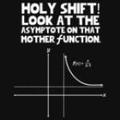1 answerLast reply by: Dr. William MurrayTue Jun 17, 2014 12:08 PMPost by Austin Cunningham on June 9, 2014How come at around 8:00, he says that x^2 is the same thing as f(x)?3 answersLast reply by: Dr. William MurrayThu Jun 5, 2014 11:43 AMPost by Govind Balaji Srinivasa Raghavan on May 29, 2014I dont know why. But all videos pause after sometime. Then it restarts again instead of continue. Also I cant skip to other part of video. Suppose tonight I watch half of video and go to sleep. Tomorrow morning, I have to watch from first, if i click on the play-head from where I should see, It automatically restarts.1 answerLast reply by: Dr. William MurrayTue Mar 4, 2014 5:00 PMPost by Damien O Byrne on February 28, 2014Does sin cos and tan formulas only apply to right angled triangles1 answerLast reply by: Dr. William MurrayWed Jan 22, 2014 3:04 PMPost by Carroll Fields on January 16, 2014In extra example I, at 3:30, why is the 'sine, cosine, and tangent of the right angle (alpha) , 1,0 , and undefined?And why for sine is it sin pi/2  ?1 answerLast reply by: Dr. William MurrayMon Oct 21, 2013 7:15 PMPost by yannick Haberkorn on October 12, 2013i have to congratulate you Dr.william murray because i actually really feel i am in a learning environment and it feels great . Much thanks1 answerLast reply by: Dr. William MurrayTue Apr 16, 2013 8:35 PMPost by Dr. William Murray on January 27, 2013Hi Emily,Good question. As Jacob says in his post above, it's because we know that the graph of f(x-c) is like the graph of f(x), but shifted c units to the right. But you have to have the negative sign in there for this to work, so when we have f(x+(something)), we write it as f(x-(-something)). Then it's clear that the shift is (-something) units to the right, that is, (something) units to the left. It's also worth reading Jacob's answer above -- same basic idea, but sometimes having a different person's phrasing helps.Thanks for taking trigonometry!Will Murray1 answerLast reply by: Dr. William MurrayTue Apr 16, 2013 8:34 PMPost by Emily Engle on January 27, 2013At 28:10 Why do you change Sin (x+ Pi/2) to Sin (x-(- Pi/2)) ?1 answerLast reply by: Dr. William MurrayFri Aug 31, 2012 5:45 PM1Post by Andraa Cram on June 25, 2012@ 21:18, why, when going in the negative direction while graphing for sine (in red), does he draw the graph as (-Pi/2,-1) instead of (-Pi/2,1)? I'm very confused by this.1 answerLast reply by: Dr. William MurraySun Jan 27, 2013 4:18 PMPost by Lourdes Johnson on June 3, 2012Why does the lecture restart around a quarter in?1 answerLast reply by: Dr. William MurraySun Jan 27, 2013 4:16 PMPost by Callistus Elue on May 23, 2012the lecture reverts to the beginning almost as soon as it starts1 answerLast reply by: Dr. William MurraySun Jan 27, 2013 4:12 PMPost by Jacob Burley on April 25, 2011At 26:33 Professor Murray gave the algebraic equation for a graph that has a constant which was f(x-c). Our equation sin(x+pi/2) has a positive where the negative is in the original equation. In order to get the correct sign there we must change the + sign into two - signs because two negatives make a positive. I know I'm not the greatest at explaining things but hopefully this helps a little bit.1 answerLast reply by: Dr. William MurraySun Jan 27, 2013 4:10 PM3Post by Shannon Bryington on February 28, 2011At 27:40 on the video: Why was x + pi over 2 changed to x - neg pi over 2?1 answerLast reply by: Dr. William MurraySun Jan 27, 2013 3:59 PMPost by Santhini Dheenathayalan on January 19, 2011Great!

### Sine and Cosine Functions

Main definitions and formulas:

• When you draw an angle θ (measured in radians) in standard position (i.e. starting on the positive x-axis), the coordinates of its terminal side on the unit circle are the cosine and sine of θ .
• Master formula for right triangles: SOHCAHTOA!
 sinθ = opposite sidehypotenuse cosθ = adjacent sidehypotenuse tanθ = opposite sideadjacent side
• A function f is odd if f(−x) = −f(x), or equivalently, its graph has rotational symmetry around the origin.
• A function f is odd if f(−x) = f(x), or equivalently, its graph has mirror symmetry across the y-axis.

Example 1:

Find the cosine and sine of 0, (π/2), π , (3π/2), and 2π .

Example 2:

Draw graphs of the cosine and sine functions. Label all zeroes, maxima, and minima.

Example 3:

A right triangle has short sides of lengths 3 and 4. Find the sine, cosine, and tangent of all angles in the triangle.

Example 4:

Graph the functions f(x) = sin(x + (π /2)) and g(x) = cos(x − (π/2)). For each one, determine if the function is odd, even, or neither.

Example 5:

A right triangle has one leg of lengths 5 and hypotenuse of length 13. Find the sine, cosine, and tangent of all angles in the triangle.

Example 6:

Graph the functions f(x) = sin(x −(π /2)) and g(x) = cos(x + (π /2)). For each one, determine if the function is odd, even, or neither.

### Sine and Cosine Functions

A right triangle has short sides of lengths 7 and 8. Find the sine, cosine, and tangent of all the angles in the triangle.
• Recall: SOHCAHTOA sinθ = [Opposite/Hypotenuse], cosθ = [Adjacent/Hypotenuse], tanθ = [Opposite/Adjacent]
• First find the length of the longest side by using the Pythagorean Theorem: a2 + b2 = c2
• 72 + 82 = x2 ⇒ 49 + 64 = x2 ⇒ 113 = x2 ⇒ √{113} = x
• Set up a triangle to help you determine which side will be the opposite and which side will be the adjacent according to your reference angle.
•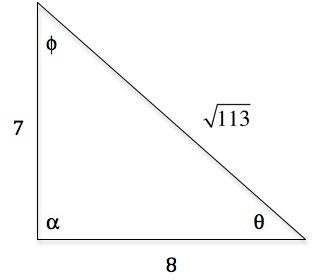sinθ = [7/(√{113} )] = [(7√{113} )/113], sinϕ = [8/(√{113} )] = [(8√{113} )/113], sinα = sin[(π)/2] = 1

cosθ = [8/(√{113} )] = [(8√{113} )/113], cosϕ = [7/(√{113} )] = [(7√{113} )/113], cosα = cos[(π)/2] = 0

tanθ = [7/8], tanϕ = [8/7], tanα = tan[(π)/2] = undefined
A right triangle has one leg of length 9 and hypotenuse of length 12. Find the sine, cosine, and tangent of all angles in the triangle.
• Recall: SOHCAHTOA sinθ = [Opposite/Hypotenuse], cosθ = [Adjacent/Hypotenuse], tanθ = [Opposite/Adjacent]
• First find the length of the longest side by using the Pythagorean Theorem: a2 + b2 = c2
• x2 + 92 = 122 ⇒ x2 + 81 = 144 ⇒ x2 = 63 ⇒ x = √{63}
• Set up a triangle to help you determine which side will be the opposite and which side will be the adjacent according to your reference angle.
•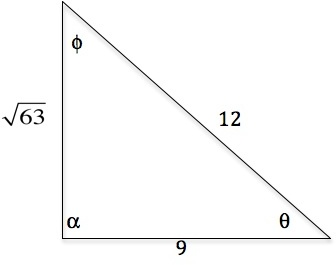sinθ = [(√{63} )/12], sinϕ = [9/12], sinα = sin[(π)/2] = 1

cosθ = [9/12], cosϕ = [(√{63} )/12], cosα = cos[(π)/2] = 0

tanθ = [(√{63} )/9], tanϕ = [9/(√{63} )] = [(9√{63} )/63], tanα = tan[(π)/2] = undefined
Use the following right triangle to find the sine, cosine, and tangent of all the angles in the triangle:
•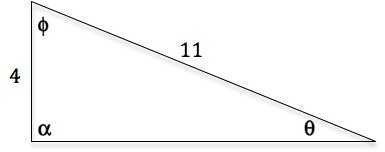• Recall: SOHCAHTOA sinθ = [Opposite/Hypotenuse], cosθ = [Adjacent/Hypotenuse], tanθ = [Opposite/Adjacent]
• First find the length of the longest side by using the Pythagorean Theorem: a2 + b2 = c2
• x2 + 42 = 112 ⇒ x2 + 16 = 121 ⇒ x2 = 105 ⇒ x = √{105}
sinθ = [4/11], sinϕ = [9/12], sinα = sin[(π)/2] = 1

cosθ = [(√{105} )/11], cosϕ = [(√{63} )/12], cosα = cos[(π)/2] = 0

tanθ = [4/(√{105} )] = [(4√{105} )/105], tanϕ = [(√{105} )/4], tanα = tan[(π)/2] = undefined
Use the following right triangle to find the sine, cosine, and tangent of all the angles in the triangle:
•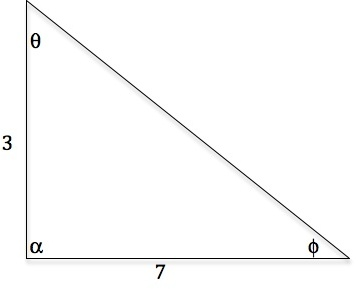• Recall: SOHCAHTOA sinθ = [Opposite/Hypotenuse], cosθ = [Adjacent/Hypotenuse], tanθ = [Opposite/Adjacent]
• First find the length of the longest side by using the Pythagorean Theorem: a2 + b2 = c2
• 32 + 72 = x2 ⇒ 9 + 49 = x2 ⇒ 58 = x2 ⇒ x = √{58}
sinθ = [7/(√{58} )] = [(7√{58} )/58], sinϕ = [3/(√{58} )] = [(3√{58} )/58], sinα = sin[(π)/2] = 1

cosθ = [3/(√{58} )] = [(3√{58} )/58], cosϕ = [7/(√{58} )] = [(7√{58} )/58], cosα = cos[(π)/2] = 0

tanθ = [7/3], tanϕ = [3/7], tanα = tan[(π)/2] = undefined
Graph the functions f(x) = sin (x − [(π)/4]) and g(x) = cos (x + [(π)/4]). Identify the zeros, maxima, and minima.
• First start off by graphing f(x) = sin(x)
•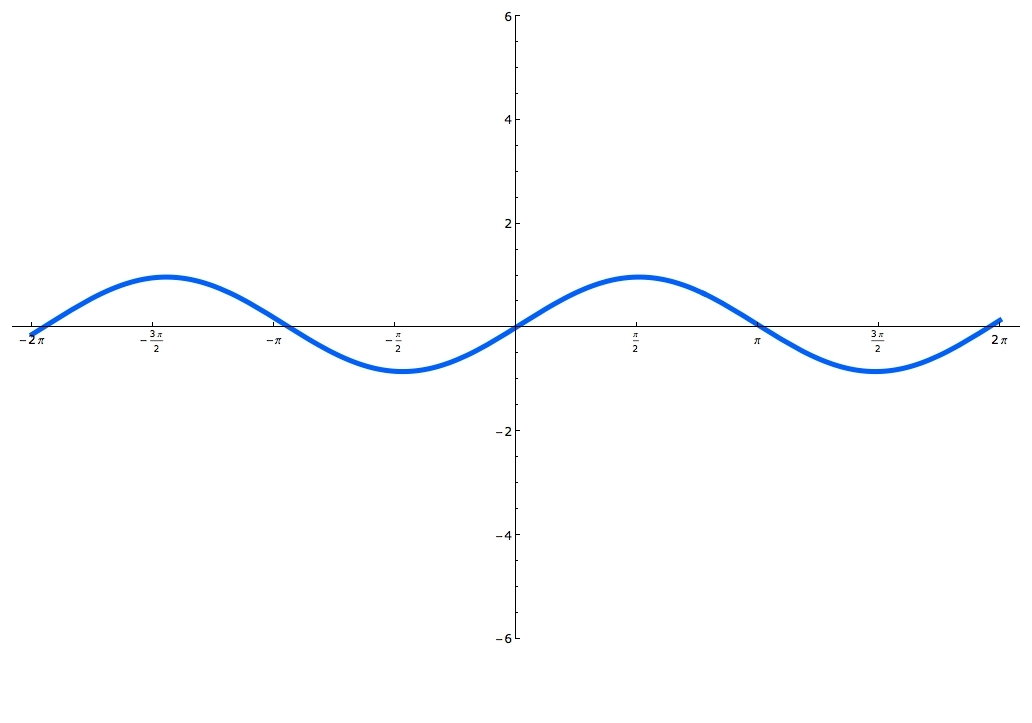• Notice that for this problem f(x) = sin(x) shifts [(π)/4] units to the right in f(x) = sin (x − [(π)/4])
•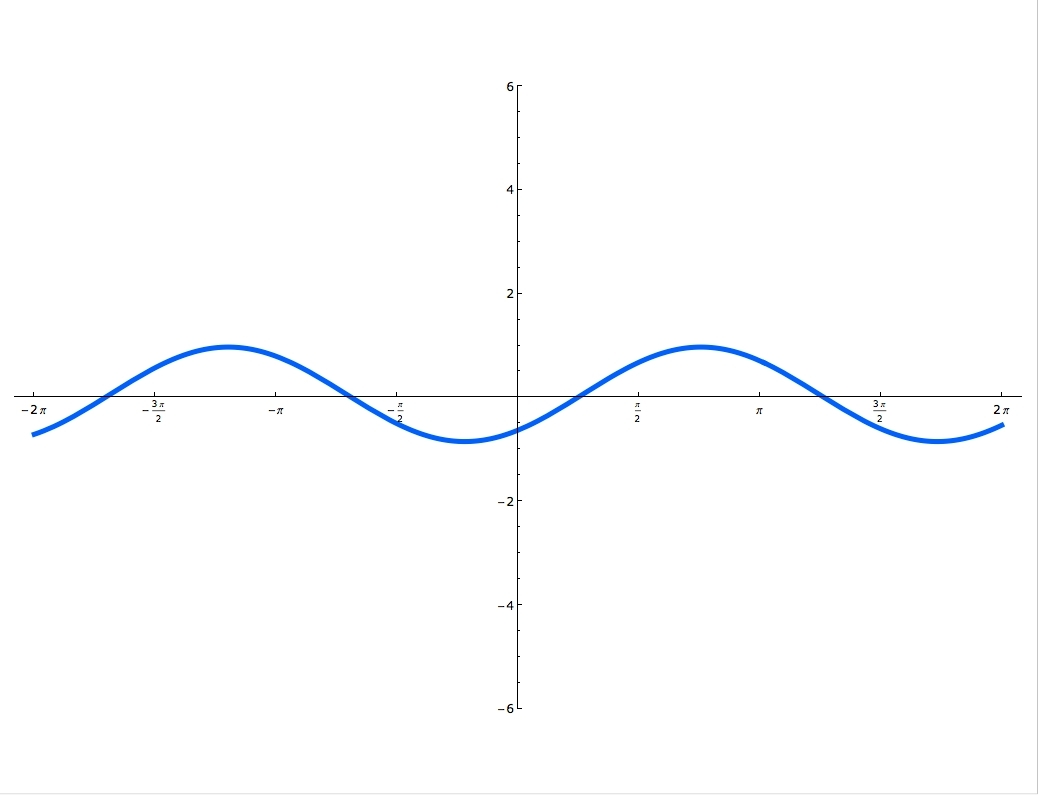• The zeroes are the points in which the function crosses the x - axis, the maxima are the highest points, the minima are the lowest points
• Now, graph g(x) = cos(x)
•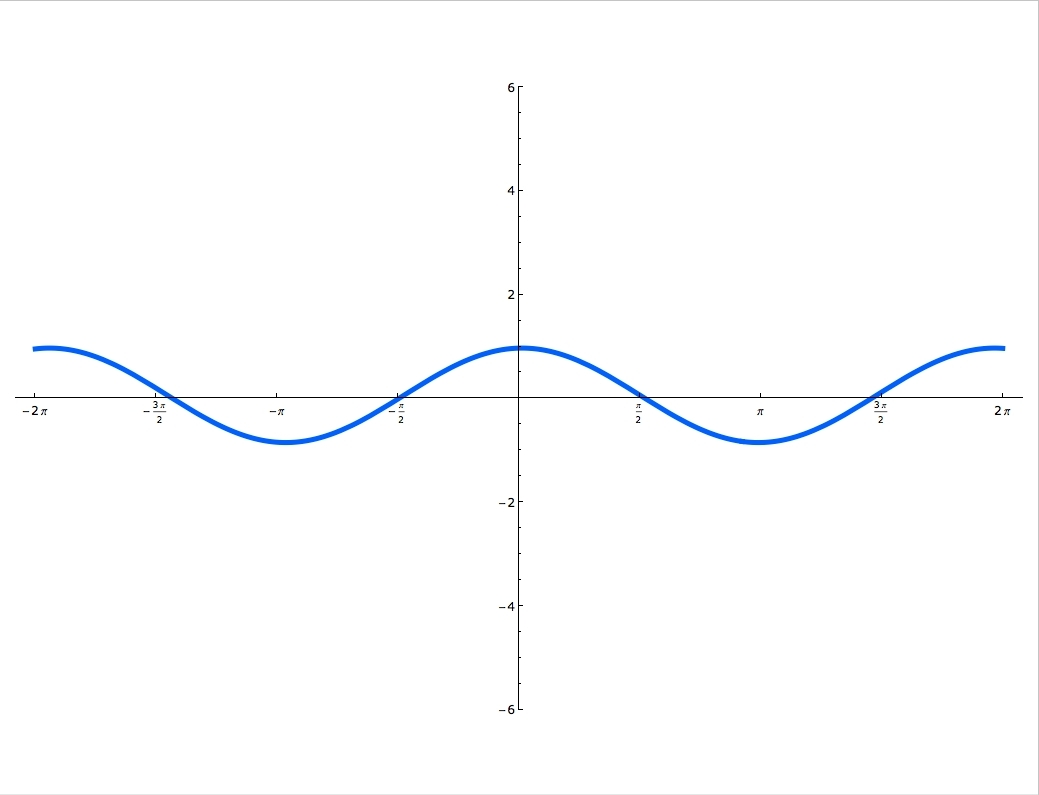• Notice that g(x) = cos(x) shifts [(π)/4] units to the left in f(x) = cos(x + [(π)/4])
•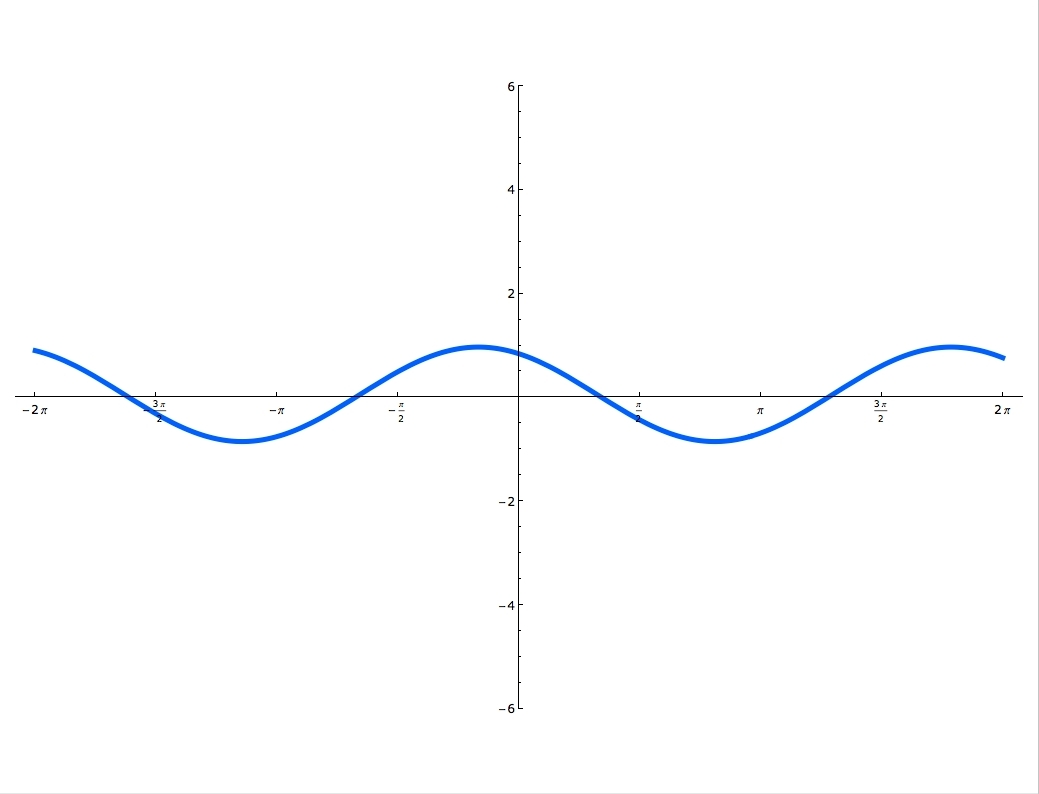f(x) = sin (x − [(π)/4]) zeroes are ([(π)/4],0), ([(5π)/4],0), ([(9π)/4],0), maxima is ([(3π)/4],1) minima is ([(7π)/4], − 1)

g(x) = cos(x + [(π)/4]) zeroes are ([(π)/4],0), ([(5π)/4],0), maxima is ( − [(π)/4],1), ([(7π)/4],1) minima is ([(3π)/4], − 1)
Graph the functions f(x) = sin (x + π) and g(x) = cos (x − π). Identify the zeros, maxima, and minima.
• First start off by graphing f(x) = sin(x)
•• Notice that for this problem f(x) = sin(x) shifts π units to the left in f(x) = sin (x + p)
•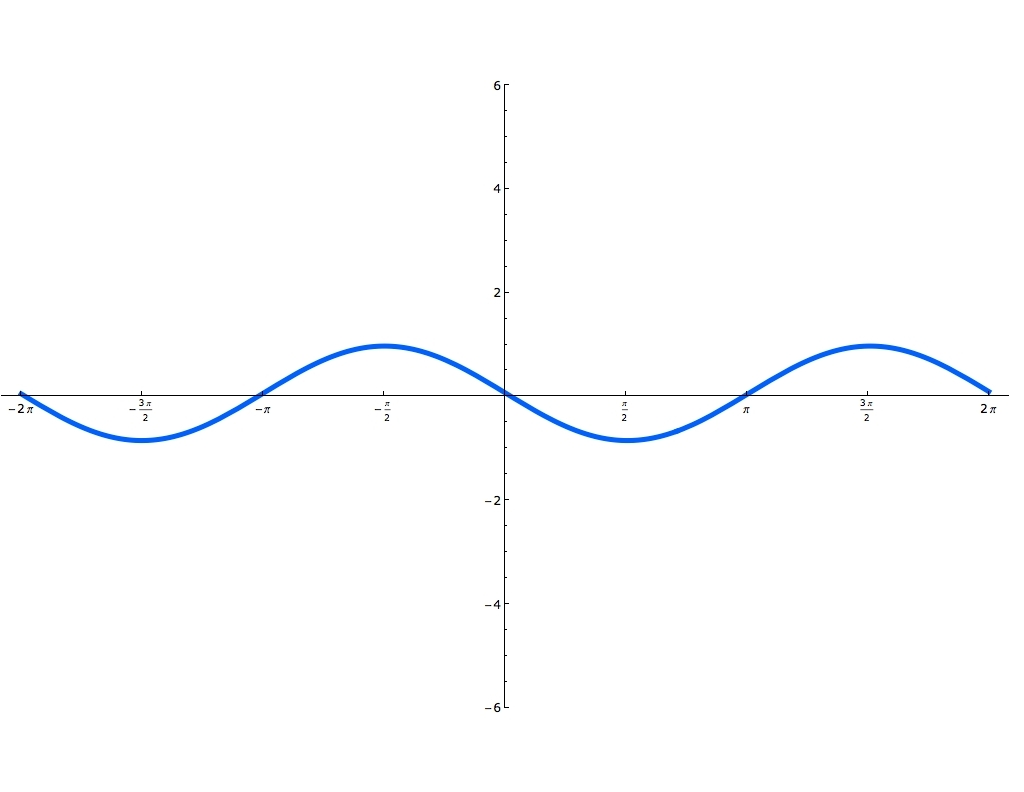• The zeroes are the points in which the function crosses the x - axis, the maxima are the highest points, the minima are the lowest points
• Now, graph g(x) = cos(x)
•• Notice that g(x) = cos(x) shifts π units to the right in f(x) = cos(x − π)
•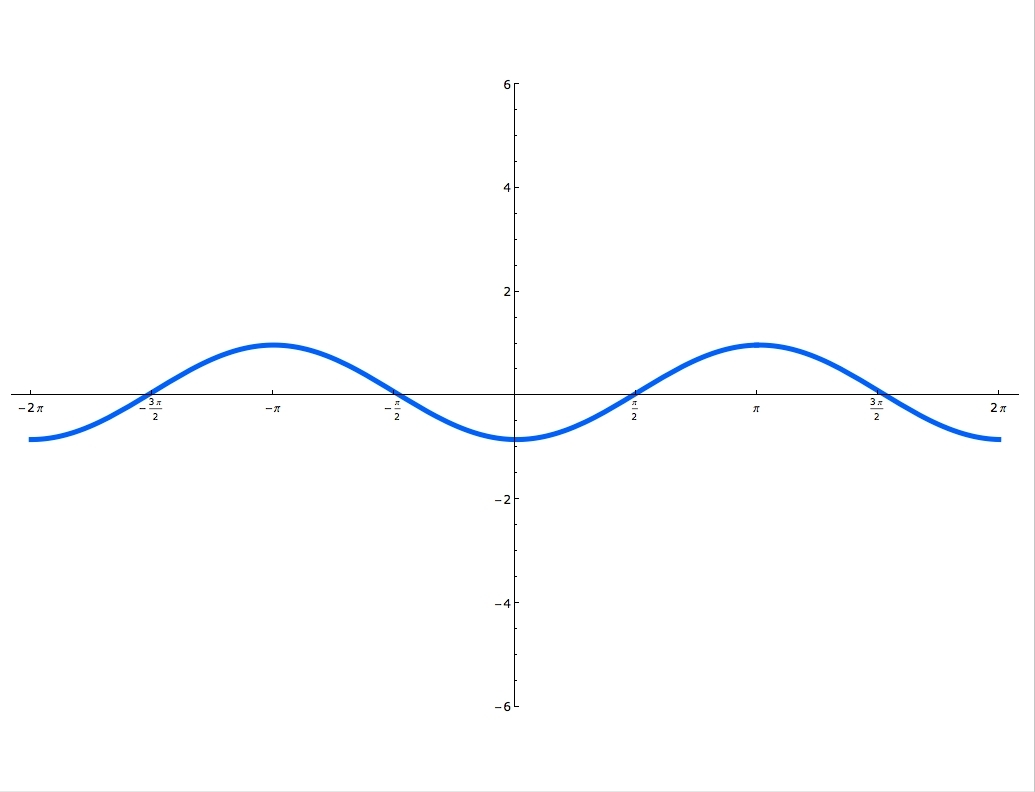f(x) = sin (x + π) zeroes are ( − π,0), (0,0), (π,0), maxima is ( − [(π)/2],1) minima is ([(π)/2], − 1)

It is an odd function

g(x) = cos(x − π) zeroes are ( − [(π)/2],0), ([(π)/2],0), ([(3π)/2],0), maxima is (π,1), ( − π,1) minima is (0, − 1), (2π, − 1)
Use the following graph to identify the zeroes, maxima, and minima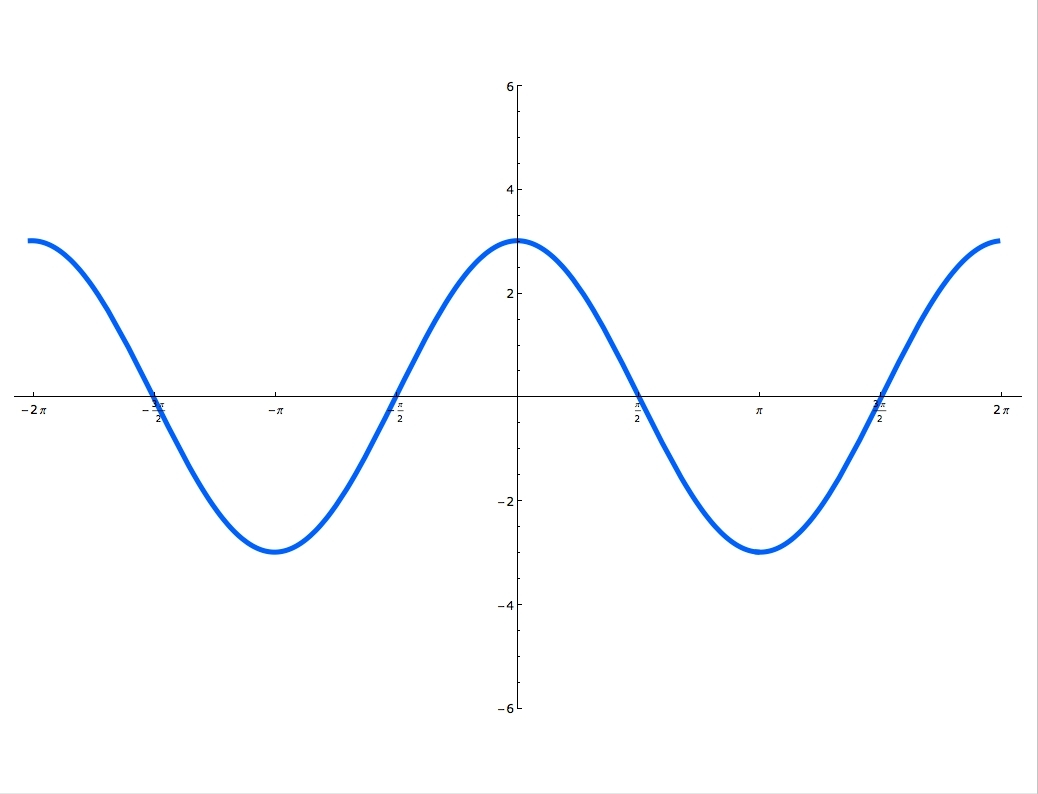• The zeroes are the points in which the function crosses the x - axis, the maxima are the highest points, the minima are the lowest points
Zeroes ( − [(3π)/2],0), ( − [(π)/2],0), ([(π)/2],0), ([(3π)/2],0)

Maxima ( − 2π, 3), (0, 3), (2π, 3)

Minima ( − π, − 3), (π, − 3)
Graph the functions f(x) = sin (x − [(π)/3]) and g(x) = cos (x + [(π)/3]). Identify the zeros, maxima, and minima.
• First start off by graphing f(x) = sin(x)
•• Notice that for this problem f(x) = sin(x) shifts [(π)/3] units to the right in f(x) = sin (x − [(π)/3])
•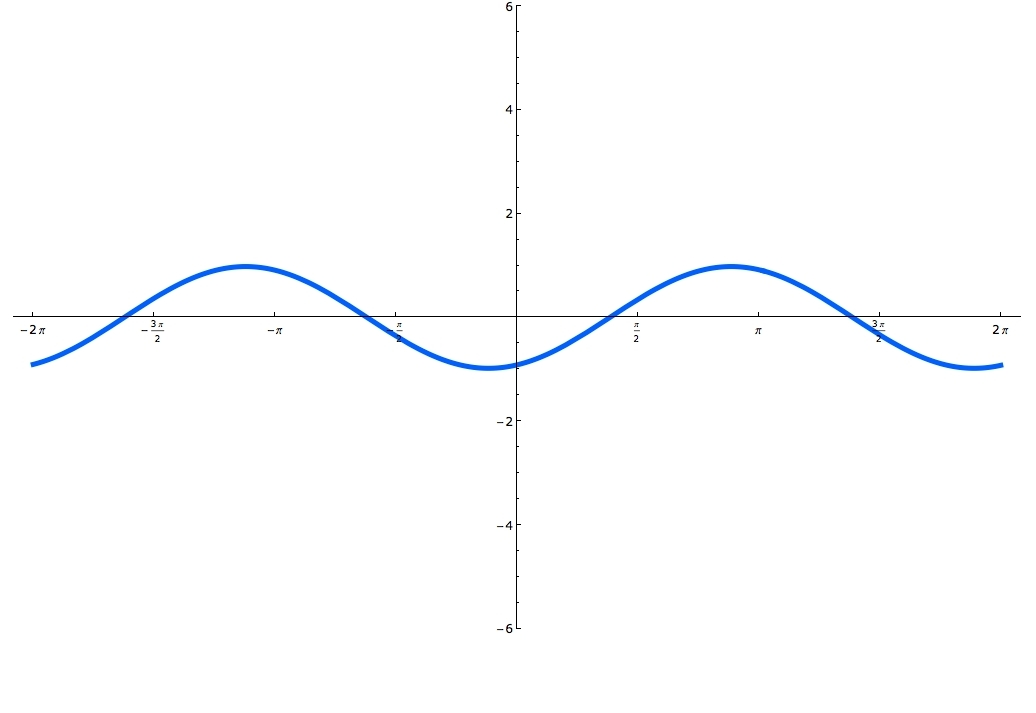• The zeroes are the points in which the function crosses the x - axis, the maxima are the highest points, the minima are the lowest points
• Now, graph g(x) = cos(x)
•• Notice that g(x) = cos(x) shifts [(π)/3] units to the left in f(x) = cos(x + [(π)/3])
•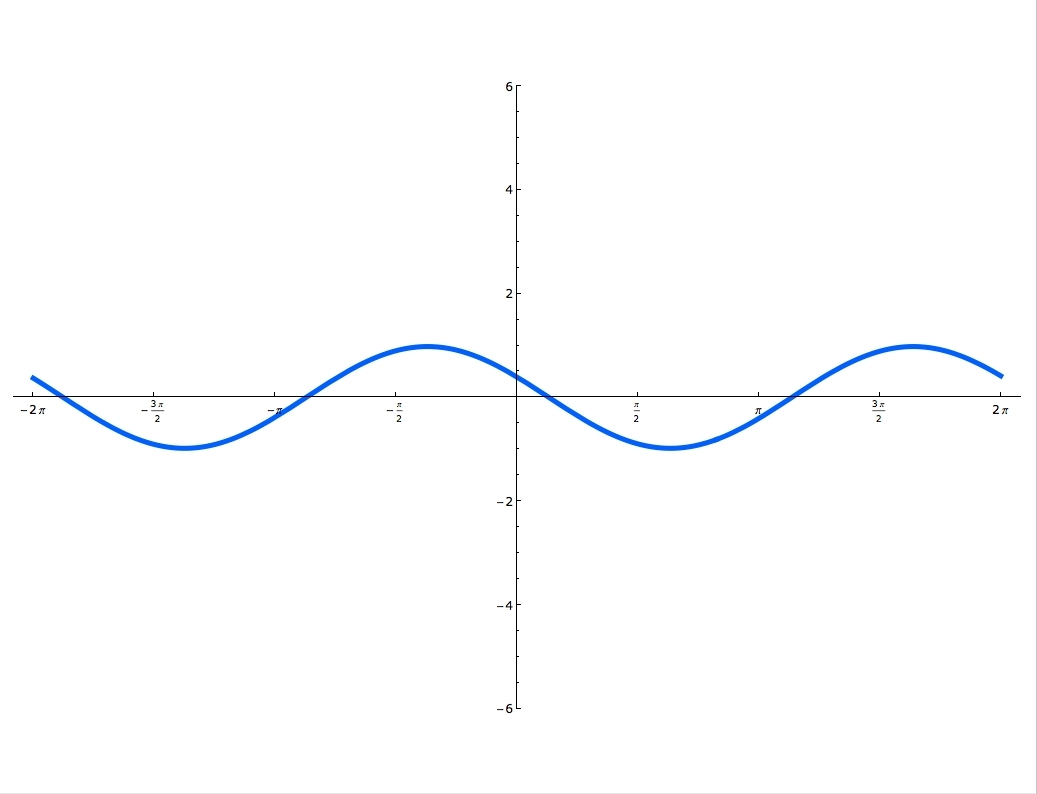Zeroes: ([(π)/6],0),([(7π)/6],0), Maxima: ( − [(π)/3],1),([(5π)/3],1), Minima: ([(2π)/3], − 1)
Graph the functions f(x) = sin (x − π) and g(x) = cos (x + π). Identify the zeros, maxima, and minima.
• First start off by graphing f(x) = sin(x)
•• Notice that for this problem f(x) = sin(x) shifts p units to the right in f(x) = sin (x − π)
•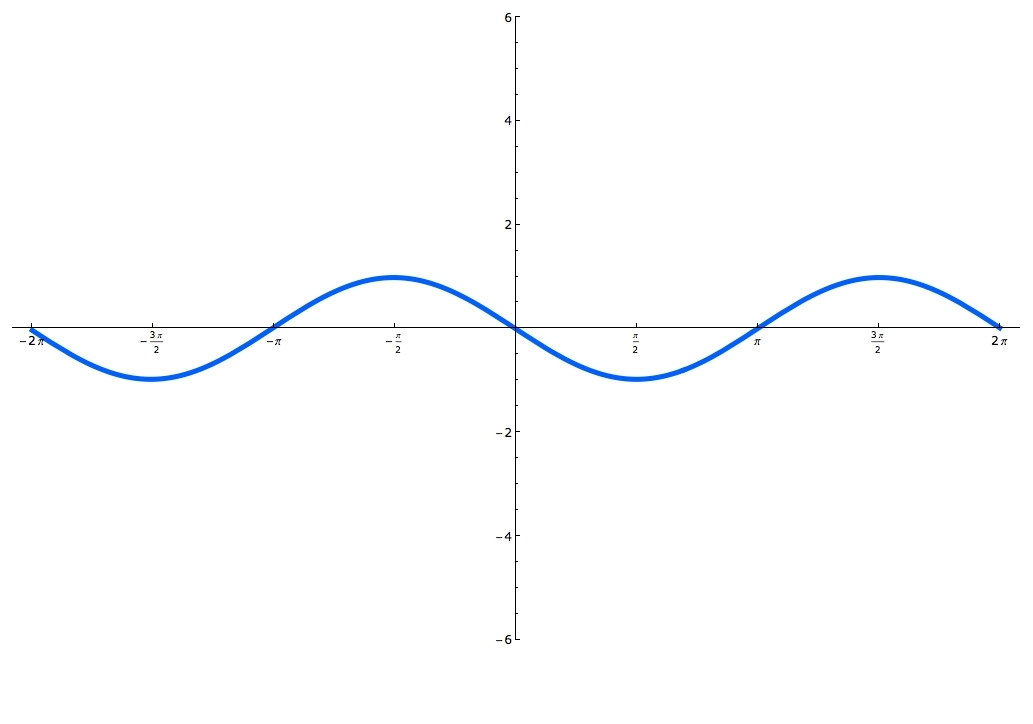• The zeroes are the points in which the function crosses the x - axis, the maxima are the highest points, the minima are the lowest points
• Now, graph g(x) = cos(x)
•• Notice that g(x) = cos(x) shifts π units to the left in f(x) = cos(x + π)
•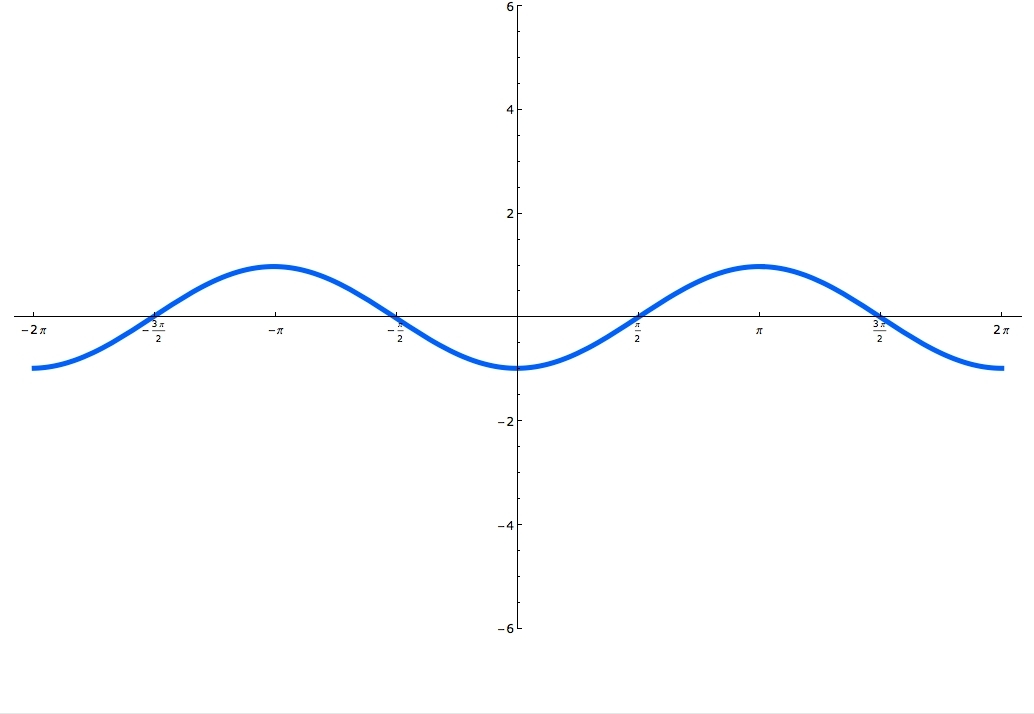Zeroes: ( − [(π)/2],0), ([(π)/2],0), ([(3π)/2],0), Maxima: ( − π, 1), (π, 1), Minima: (0, − 1), (2π, − 1)
Use the following graph to identify the zeroes, maxima, and minima
•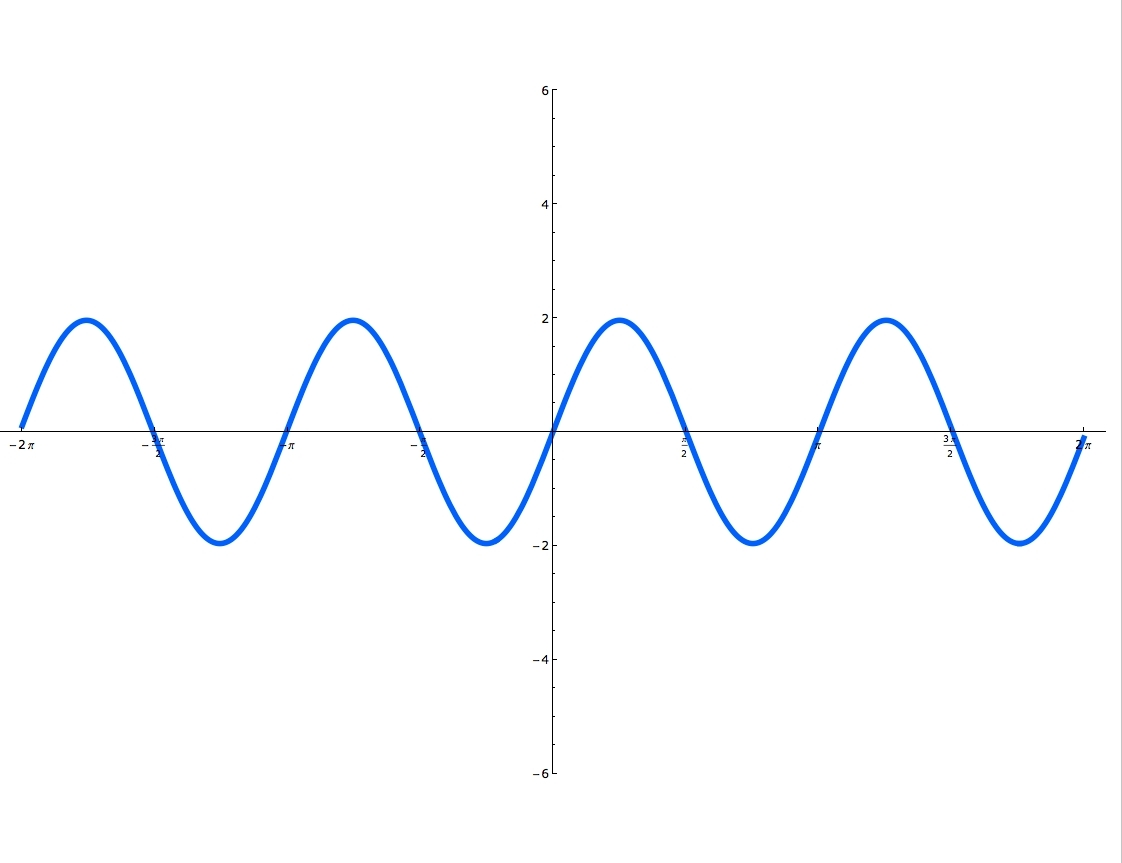• The zeroes are the points in which the function crosses the x - axis, the maxima are the highest points, the minima are the lowest points
Zeroes ( − 2π,0), ( − [(3π)/2],0), ( − π,0), ( − [(π)/2],0), (0,0), ([(π)/2],0), ([(3π)/2],0), (2π,0)

Maxima ( − [(7π)/4], 2), ( − [(3π)/4], 2), ([(3π)/4], 2), ([(7π)/4],2)

Minima ( − [(5π)/4], − 2), ( − [(π)/4],2), ([(π)/4],2), ([(5π)/4], − 2)

*These practice questions are only helpful when you work on them offline on a piece of paper and then use the solution steps function to check your answer.

### Sine and Cosine Functions

Lecture Slides are screen-captured images of important points in the lecture. Students can download and print out these lecture slide images to do practice problems as well as take notes while watching the lecture.

• Intro 0:00
• Sine and Cosine 0:15
• Unit Circle
• Coordinates on Unit Circle
• Right Triangles
• Master Right Triangle Formula: SOHCAHTOA
• Odd Functions, Even Functions 4:40
• Example: Odd Function
• Example: Even Function
• Example 1: Sine and Cosine 10:27
• Example 2: Graphing Sine and Cosine Functions 14:39
• Example 3: Right Triangle 21:40
• Example 4: Odd, Even, or Neither 26:01
• Extra Example 1: Right Triangle
• Extra Example 2: Graphing Sine and Cosine Functions

### Transcription: Sine and Cosine Functions

Ok we are going to work on some extra examples with you, I hope you have time to practice a little bit on your own.0000

Here we are given a right triangle that has 1 leg of length 5, and a hypotenuse of length 13.0006

What we want to do is find the sin, cos, and tan of all the angles in the triangle.0024

I hope you know that the master formula here is going to be SOHCAHTOA.0029

That is the one we are going to use but in order to use SOHCAHTOA you have to know the lengths of all the sides of the triangle.0035

Let me set a variable for this length, we need to solve x2 + 52 = 132.0044

That is x2 + 25 =169, x2 = 169-25, that is 144, so x=12.0057

That tells us our third side length, it looks like my triangle is not really drawn on the scale because 12 is a lot bigger than 5.0073

But we can still do the calculations here even if we did not draw the triangle perfectly to scale, you can still figure out the calculations.0079

Let me label the angles (theta) and (phi) and we will look at (theta) first.0088

Sin(theta) using SOHCAHTO is opposite/hypotenuse, so that is 12 is the opposite angle of (theta) and the hypotenuse is 13.0094

Cos(theta)=adjacent of the side is 5/13, the tan(theta) =opposite of adjacent that is 12/5.0112

Sin(phi) let us do that one first, is equal to the opposite/hypotenuse.0134

The opposite side to (phi) is 5 and the hypotenuse is still 13.0146

The cos(phi) is equal to the adjacent side, the side adjacent to (phi) is 12/13.0155

The tan(phi) is equal to the opposite of adjacent, the opposite side of (phi) is 5, and the adjacent side is 12.0165

Finally, let me label the right angle (alpha), the sin(alpha), I’m not going to use SOHCAHTOA on this but the sin (alpha) is just the sin (pi/2) is 1.0180

The cos(alpha) is the cos (pi/2) which is 0 and then the tan(alpha).0194

Again we have not really learned about tan yet, but it turns out that the tan of a 90 degree angle or the tan of pi/2 is undefined.0202

I learned why that is true when we get to studying the tan function later on.0211

To recap here, everything came out of the master formula SOHCAHTOA, memorize that word SOHCAHTOA or memorize the phrase Some Old Horse Caught Another Horse Taking Oats Away.0225

That helps you to remember how to figure out all these sin, cos, and tan, once you know the lengths of the sides of the right angle.0237

Our last example here is two functions that we need to graph, two modified sin waves and remember that the way to do that is to start out with sin and cos graphs that you know.0000

Let me start graphing sin(x), there is pi, 2pi, 1, -1, pi/2, 3pi/2.0012

Remember that sin(x) starts at 0, goes up to 1, comes back to 0 down to -1.0035

What I graphed there in black is sin(x), now sin(x)-pi/2 I will do that in blue.0052

Sin(x)-pi/2 that takes the graph and it shifts it over to the right by pi/2 units.0060

I’m going to take this graph in black and shift it over to the right by pi/2 units.0067

Let me extend that a little bit so I will know how to shift it, now I’m going to shift it over to the right by pi/2 units.0077

Now it starts at 0 to pi/2, comes back to 0 to 3pi/2 down to -1, back to 0.0082

What I have drawn there in blue is sin(x) – pi/2, it is just a basic sin graph but moved over by pi/2 units.0104

We have to figure out if its odd, even, or neither0113

Remember that odd has rotational symmetry, even has mirror symmetry across the y axis.0116

Clearly this blue graph that I have drawn here has mirror symmetry across the y axis but not rotational symmetry.0124

This is an even function because it has mirror symmetry across the y axis.0135

Let us move on to g(x), that is cos, again I will start with the cos graph that I have memorized and hopefully you have two.0151

There is pi, 2pi, 3pi/2, pi/2, 0, -pi, -pi/2, 1 and -1, that is a little low, let me draw that a little higher, -1.0163

Remember the cos graph starts at 1 goes down to 0, down to -1 at pi and comes back to 0 and back 1 at 2pi.0189

What I graphed there in black was cos(x), the basic cos curve, cos(x + pi/2), I will do this in red.0210

You want to think about that as cos(x - -pi/2) and I do that to create that negative sign because when you have a shift you always want to have a negative sign there.0222

It helps you figure out which way it is shifting, that means shifting –pi/2 units to the right, which means pi/2 units to the left.0235

I take this graph and I shift it p/2i units to the left, so that means it will start here go down to 0, down to -1, back to 0 up to 1 and back to 0.0244

That was a little bit of too high there, that curve in red is cos(x + pi/2) and if we look at that curve it has rotational symmetry around the origin.0264

If you rotate it back graph, 180 degrees around the origin it would look the same.0286

It definitely does not have a mirror symmetry around the y axis, so this is an odd function.0292

Odd functions have rotational symmetry around the origin, that is an odd function.0303

Remember the way to check whether they are odd or even is to check which kind of symmetry they have or maybe they do not have either kind of symmetry.0310

That is the end of our lessons on sin and cos, this is www.educator.com.0320

Hi, this is educator.com and we are here to talk about sine and cosine functions.0000

I'll start out by giving you the definitions and kind of the master formulas.0007

Then we'll go through and work on a bunch of examples.0010

The definition of sine and cosine of an angle is, you start out with the axis and the unit circle it's important to know that.0015

This is a unit circle meaning the radius is 1.0029

What you do is you draw that angle in standard position, meaning it has one side on the x-axis.0033

There is data right there.0042

Then you look at the coordinates of the point, the x and y coordinates on the unit circle.0044

The x-coordinate is, I'll go over that in red, that's the x coordinate right there.0051

The y-coordinate, that's the one in blue.0060

Those coordinates are, by definition, the...0063

I want to do the cosine, in red, cos(θ) and then, in blue, sin(θ).0071

That's the definition of what an angle is in terms of coordinates on the unit circle.0083

If you know what the angle is, you try to figure out what its x-coordinate and its y-coordinate are.0090

Let's call these cos(θ) and the sin(θ).0097

Now, we'll see some more examples of that later so that you'll know how to actually compute the cosine and sine, but the definition just refers to those coordinates.0101

The most common use of sine and cosine probably is in terms of right triangles.0113

Let me draw a right triangle.0120

Right triangle just means a triangle where one of the angles is a right angle, a 90-degree angle, or in terms of radians, π over 2.0123

What you do is, you let θ be one of the angles that is not the 90-degree angle, so one of the other angles.0130

Then you label each one of the sides in terms of its relationship to θ.0139

The one next to θ is called the adjacent side.0145

The one opposite θ is called the opposite side.0151

The long side is called the hypotenuse.0161

Then we have the master formula for right triangles, which is, the sine of θ is equal to the length of the opposite side divided by the hypotenuse.0168

The cosine of θ is equal to the adjacent side divided by the hypotenuse.0181

The tangent of θ, which is something we haven't officially defined yet, so we'll learn about tangent in a later lesson.0185

I just want to give you the right triangle formula now, because we're going to try to remember them all together.0193

The tangent of θ when we get to it will be the opposite side divided by the adjacent side.0200

We don't want to worry too much about tangent now because we haven't learned about it in detail yet.0205

I'll get to those later.0209

If you put all these formulas together, it's kind of hard to remember their relationships.0211

So people have come up with this acronym.0217

Sine is equal to opposite over hypotenuse.0221

Cosine is equal to adjacent over hypotenuse.0225

Tangent is equal to opposite over adjacent.0227

If you kind of read that quickly, people call it SOH CAH TOA.0233

If you talk to any trigonometry teacher in the world, or any trigonometry student in the world, they should have heard the word SOH CAH TOA,0235

because that's kind of the master formula that helps you remember all these relationships.0242

They're kind of hard to remember on their own, but if you remember SOH CAH TOA, you won't go wrong.0247

If you have trouble remembering that, there is a little mnemonic device that people also use.0253

Some old horse caught another horse taking oats away.0262

If you remember that sentence, if that's easier for you to remember than SOH CAH TOA, then you can remember all these formulas.0268

There is another definition that we need to learn which is, that a function is odd if f(-x) is equal to -f(x).0276

Let's figure out what that means.0289

We're going to talk about odd and even function.0291

Let me give you an example here.0294

F(x) is equal to x3.0299

Well, let's try f(-x) here.0305

I'm going to check this definition of odd function here.0307

So, f(-x), that means you put -x into the function, so that's (-x)3 which is (1)3 times x3,0310

(-1)3 is -1, so that's just -x3, and that's negative of the original f(x).0326

For x3, f(-x) is equal to -f(x), which means it's an odd function.0334

There is a way to check this graphically.0342

If you graph f(x)=x3, it looks something like this.0348

That means that if you look at a particular value of x, and you look at f(x) there, and then you look at -x, f(-x)=-f(x).0355

That's what it looks like graphically.0379

What it means is that the graph has what I call rotational symmetry around the origin.0383

If you put a big dot on the origin and if you spun this graph around 180 degrees, it would look the same.0393

That's because f(x) and f(-x) being opposites of each other.0400

If you spin the graph around 180 degrees and it looks the same, if it looks symmetric with itself, that's called an odd function.0408

The way you remember that odd functions have that property is, just remember x3, x to the third, because 3 is an odd number and x3 is an odd function.0417

Something, kind of, has that property that x3 has, then it's an odd function.0428

They're companion definition to that is that f is even if f(-x) equals f(x).0435

The difference there was that negative sign on the odd definition.0443

No negative sign here on the even definition.0447

Let me give you an example of an even one.0450

Let's define f(x) to be x2.0456

Well, f(-x) is equal to, you plugin -x into the function, so (-x)2 well that's just the same as x2, which is the same as the original f(x).0462

So, f(-x) is equal to f(x), that checks the definition, so it's even.0478

Of course you'll notice that x2, the 2 there is an even number, that's no coincidence.0486

That's why we call even functions even is because they sort of behave like x2.0494

If you graph those, let me graph x2 for you.0500

That's a familiar parabola that you learned how to graph in the algebra section.0505

If you take a value of x, and look at f(x),0511

then you take f(-x), f(-x) is not -f(x), it's f(x) itself.0517

It's the same value as f(x) itself.0532

You get this f(-x) is equal to f(x).0537

What that means is that you have a, kind of, symmetry across the y-axis with even functions.0542

If a function is symmetric across the y-axis, if it looks like a mirror image of itself across the y-axis, then that's an even function.0555

That's why I say it has mirror symmetry across the y-axis.0567

That's what an even function looks like.0572

There is a common misconception among students.0575

People think, well with numbers, every number's either odd or even.0578

People think, well, every function is odd or even and that's not necessarily true.0583

Just for example, here's a line but that is not either0590

that does not have a rotational symmetry around the origin nor does it have mirror symmetry around the y-axis.0600

That function, this line, is not an add or an even function.0607

It's a little misleading people think every function has to be an odd or an even function.0612

That's not true.0616

It's just true that some functions are odd, some functions are even, some functions are neither one.0618

We'll practice some examples of that.0624

First, we're going to look at some common angles and we're going to figure out what the cosines and sines are.0626

Let me draw a big unit circle here.0635

That's a circle of radius 1.0646

Let's remember where these angles are, 0, of course is on the positive x-axis.0648

Here's the x-axis, here's the y-axis, there is zero,0655

π over 2, remember that's the same as a 90-degree angle, that's a right angle, so that's up there π over 2;0660

π is over here, that's a 180 degrees;0666

3π over 2, is down here, and 2π is right here.0671

We want to find the cosine and sine of each one of those angles.0676

Now remember, cosine and sine, by definition, are the x and y coordinates of those angles.0680

What are these x and y coordinates?0689

The 0 angle, it's x-coordinate is 1, and it's y-coordinate is 0.0691

That tells us that cos(0) is 1, and that sin(0) is 0.0698

Pi over 2 is up here, and so it's cosine is the x-coordinate,0712

well, the x-coordinate of that point is 0.0726

The y-coordinate is 1, and so that's the sin of π over 2.0730

Pi is over here at (-1,0), so that's the cosine and sine of π.0739

Cos(π) is -1, sin(π) is 0.0748

Finally, 3π over 2 is down here at (0,-1), so that's the cosine and sine of 3π over 2.0760

Cos(0), sin(π/2), is -1.0772

And one more, 2π is back in the same place as 0, so it has the same cosine and sine.0783

Cos(2π) is 1, sin(2π) is 0.0790

That's how you figure out the cosines and sines of angles.0805

As you graph them on the unit circle, and then you look at the x and y coordinates.0808

The x-coordinate is always the cosine, and the y-coordinate is always the sine.0813

By the way, these are very common values, 0, π/2, 3π/2, and 2π.0819

You should really know the sines and cosines of these angles by heart.0826

They come up so often in trigonometry context that it's worth memorizing these things, and being able to sort of regurgitate them very very quickly.0830

If you ever forget them though, if you ever can't quite remember what the cosine of π/2, or the sine of 3π/2 is,0841

Then, what you do is draw yourself a little unit circle, and you figure what the x and y coordinates are and you can always work them out.0849

It's worth memorizing them to know them quickly, but if you ever get confused, you are not quite sure, just draw yourself a unit circle and you'll figure them out quickly.0857

We're going to use these values, so I hope you will remember these values for the next example.0869

In the next example, we're being asked to draw the graphs of the cosine and the sine functions, so let's remember what those values are.0879

We're to label all the zeros, and the maxima and the minima of these functions.0888

Let me set up some axis here.0892

I'm going to label my x-axis in terms of multiples of π.0898

The reason I'm going to do that is because we're talking about cosines and sines of multiples of π.0905

That's π, that's 2π, that's π/2, and that's 3π/2.0915

That's 0, of course.0929

The y-axis, I'm going to label as 1 and -1.0931

I've set up my scale here, remember that π is about 3.14 so it's a little bit bigger than 3.0940

I've set up my scale here so that the π is about a little bit beyond 3 units on the graph.0945

I'll extend it a little bit on the left here as well.0952

We've got -π, I'll draw that around -3 and -π/2.0955

I want to graph the sine and cosine function according to those values that we figured out.0963

Remember that the sine and cosine function are correspond to the coordinates of angles on the unit circle.0968

So sine and cosine,remember, are the x and y coordinates of angles on the unit circle.0978

Now, those coordinates will never get bigger than 1 or smaller than -1.0986

That's why on my y-axis, I only went up to -1 and 1, because the coordinates will never get bigger than -1 and 1.0994

Let me start out with the cosine function.1004

I'll do that one in blue, y=cos(x).1007

We'll remember the values that we learned in the previous question.1014

Cos(x), cos(0) is 1, cos(π/2) was 0, cos(π) is -1, cos(3π/2) is 0 and cos(2π) is 1.1018

What you get is this smooth curve.1044

After 2π, remember, after you circle 2π radians, then everything starts repeating.1057

What happens after 2π is that it repeats itself.1065

It repeats itself in the negative direction as well.1071

Now we know what the graph of cos(x) looks like.1080

I'll do the sine graph in red.1085

Remember that sin(0) is 0, sin(π/2) is 1, sin(π) was 0 again, sin(3π/2) is -1, sin(2π) is 0.1092

I'm doing this from memory and hopefully you remember these values as well.1110

But if you can't remember these values, you know you can always look back at the unit circle and figure them out again just from their coordinates.1114

The sine graph, I'm going to connect this up into a smooth curve.1122

It repeats itself after this.1139

It repeats in the negative direction as well.1146

That's what y=sin(x) looks like.1151

It actually has the same shape as cos(x) but it's shifted over on the graph.1153

Now, we're asked to label all zeros, maxima and minima.1160

Let me go through and label the zeros first.1163

This is on the cos(x), this is (π/2,0), that's the 0 right there.1167

There is one right there, (3π/2,0).1177

This one, even though I haven't labeled it on the x-axis, is actually (5π/2,0), because it's π/2 beyond 2π,(-π/2,0).1182

Those are the zeros of the cosine graph.1197

The maxima, the high points, remember, cosine and sine never get bigger` than 1 or less than -1.1202

Any time it actually hits 1, it's a maximum.1207

They're the two maxima, at 0 and 2π.1213

The minimum value is -1, so there is (π,-1) and the next one would be at (3π,-1), there is one at (-π,-1).1218

Now, let me do the zeros of this sine graph.1240

There is one (0,0), (-π,0), (π,0), and (2π,0).1245

The maximum value would be 1 and that occurs at π/2, and again at 5π/2.1258

The minimum value would be at 3π/2, where the sine is -1,1273

Remember sine and cosine never go outside that range, -1 to 1,1278

and at -π/2.1282

All these values you should pretty much have memorized there the sort of simplest values, the easiest ones to figure out of sine and cosine.1289

Let's try an example where we're using this trigonometric functions in a triangle.1301

What we're told is that a right triangle has short sides of length 3 and 4.1307

We're asked to find the sine, cosine, and tangent of all angles in the triangle.1317

Remember, I haven't really explained what tangent is yet, but we did learn that formula SOH CAH TOA.1323

That's what we're going to be using here.1327

The first thing we need to figure out here is what the hypotenuse of this triangle is.1330

We have the Pythagorean theorem that says, h2 = 32 + 42, which is 9 plus 16, which is 25.1334

That tells us that the hypotenuse must be 5.1349

Now we're going to find the sine, cosine and tangent of each one of these angles.1354

Let's figure out this angle first, so I'll call it θ, sin(θ), remember SOH CAH TOA,1364

let me write that down for reference here, SOH CAH TOA,1372

sin(θ) is equal to opposite over adjacent1381

That's 4, that's the opposite side from θ over...1386

Sorry, I said sin(θ) is opposite over adjacent, of course, sin(θ) is opposite over hypotenuse, and the hypotenuse is 5.1394

So, sin(θ) there is 4/5.1401

Cos(θ) is equal to adjacent over hypotenuse.1404

Well, the side adjacent to θ is 3, hypotenuse is still 5.1409

Tan(θ) is equal to opposite over adjacent.1416

Again, we haven't really learned what a tangent means yet, but we can still use SOH CAH TOA.1423

The opposite over adjacent is 4/3.1427

Let me call the other angle here φ.1435

Sin(φ) is equal to the opposite over the hypotenuse, so 3/5.1442

Cos(φ) is equal to adjacent over hypotenuse, the adjacent angle beside φ is 4.1451

Tan(θ) is equal to the opposite over adjacent, so that's 3/4.1463

Finally, we have the right angle here, I'll call that α.1472

We can't really use SOH CAH TOA on that, but I know that sin(α),1476

α is a 90-degree angle, or in terms of radians, it's π/2.1480

The sin(π/2), we learned before, is 1.1489

Cos(α) is cos(π/2), and we learned that the cos(π/2) before was 0.1494

Finally, tan(α) is tan(π/2), and we haven't really learned about tangent yet.1505

In particular, we haven't learned what to do with the tan(π/2).1514

But we'll get to that in a later lecture, and we'll learn that that's actually not defined.1518

So we can't give a value to the tangent of π/2.1523

All of these angles were things we worked out just using this one master formula, SOH CAH TOA.1532

That tells you the sine, cosine and tangent of the small angles in the triangle.1541

The SOH CAH TOA does not really apply to the right angle.1548

But we already know the sine and cosine of a right angle, of a 90-degree angle, because we figured them out before.1550

We'll try some more examples here.1558

I want to try graphing the function sin(x + π/2) and cos(x - π/2).1562

Then, I want to determine whether these functions are odd or even, or neither one.1570

Well, something that's really good to remember here from your algebra class, or from the algebra lectures here on educator.com,1576

is that you have a function, and you try to graph f(x) minus a constant,1584

what that does is it moves the graph of the function over by the amount of the constant.1593

That's very useful in the trigonometric setting.1599

Let me start out by graphing f(x)=sin(x).1602

And we did that in the previous example, and I remember what the sine graph looks like.1608

It starts at 0, it peaks at π/2, it goes back to 0 at π, it bottoms out at 3π/2 at -1, and then it goes back to 0 at 2π.1615

What I graphed there was just sin(x), I have not introduced this change yet.1635

What I'm going to do, in blue now, is the sin(x + π/2).1643

What that's going to do is going to move the graph over π/2 units.1651

But remember there was a negative sign in there that I don't have here.1656

This is really like, sin[x - (-π/2)].1661

It moves the graph over -π/2 units, which means it moves it to the left π/2 units.1672

I'm going to take this graph and I'm going to move it over to the left π/2 units.1680

Now it's going to start at -π/2, come back down at π/2, bottom out at π, and come back to 0 at 3π/2.1691

So there is -π/2, 0, π/2, and it comes back at 3π/2.1709

That's what our graph of sin(x + π/2) looks like.1719

Then the question is, is that odd or even, or neither?1725

Well, remember there is a graphic way to look at the graph of a function in determining whether it's odd or even, or neither.1730

An odd function, remember, has rotational symmetry, and even function has mirror symmetry across the y-axis.1738

Well, if you look at this at this graph, it certainly does not have rotational symmetry.1750

If you tried to rotate it around the origin, it would end up down here, and that would be a different graph.1757

However, it does have mirror symmetry around the y-axis.1762

So because it's mirror symmetric around the y-axis, sin(x + π/2).1772

F(x) is an even function because it has mirror symmetry, mirror symmetry across the y-axis.1781

OK, let us move on to the next one, cos(x - π/2).1812

Again, I'm going to start with the basic cosine function that we learned how to graph in a previous example.1816

So that my mark's here, there is π, there is 2π, π/2, 3π/2, 0, -π/2.1829

Now, cosine had zero at 1, then it comes down to 0 at π/2, bottoms out at -1 at π, comes back to 0 at 3π/2, and by 2π, it's back up at 1.1841

What I have just graphed there in black is cos(x).1862

I have not tried to introduce the shift yet.1865

But what we want to do is to graph cos(x - π/2).1871

That's like saying, you see up here is π/2, that's going to shift the graph π/2 units to the right,1878

because it's -π/2, it shifts it to the right.1888

We take this graph and we move it over to the right π/2 units.1890

I drew that a little too high, let me flatten that out a little bit.1904

What we have there is the graph of cos(x - π/2), and of course that keeps going in the other direction there.1910

We see, actually if you look carefully, cos(x - π/2) actually turns out be the same graph as sin(x).1921

That's a familiar function if you remember those graphs.1930

Again, we're being asked whether the function is odd or even, or neither.1935

For odd, we're checking rotational symmetry around the origin.1939

Look at that.1944

If you rotate the graph 180 degrees around the origin, what you'll end up with is exactly the same picture.1946

g(x) is an odd function because it has rotational symmetry around the origin.1953

Is it an even function?1982

Does it have mirror symmetry around the y-axis?1984

No, it does not because it has this kind of bump on the right hand side, and it does not have the same bump on the left hand side, so it's not an odd function.1987

Sorry, it's not an even function.1996

It's just an odd function.1998

What we did there was we started with the sine and cosine graphs that we remembered.2000

it's worth memorizing the basic sine and cosine graphs.2004

Then we examined the shift that each one introduced.2008

Each one got shifted π/2 units to the right or left.2013

Then we drew the new graphs.2016

Then we looked back at them and we checked what kind of symmetry do they have.2019

Do they have rotational symmetry or mirror symmetry?2022

And that tells us whether they are odd or even.2027

OR

### Start Learning Now

Our free lessons will get you started (Adobe Flash® required).